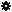﻿ Object Model: PolygonFaceCollection.NeighborEdges

PolygonFaceCollection.NeighborEdges

## PolygonFaceCollection.NeighborEdgesv3.0

### Description

Returns an collection Edge neighbors within a given distance.

### C# Syntax

 ```EdgeCollection PolygonFaceCollection.NeighborEdges( Int32 in_lDistance ); ```

### Scripting Syntax

 ```oReturn = PolygonFaceCollection.NeighborEdges( [Distance] ); ```

### Parameters

Parameter Type Description
Distance Integer Distance is an integer value representing the degree of neighborhood (eg: degree=2 for a polygon means its adjacent edges plus the adjacent edges of the adjacent polygons)

Default Value: 1

### Examples

#### VBScript Example

 ```set oCube = ActiveSceneRoot.AddGeometry( "Cube", "MeshSurface" ) set oGeometry = oCube.ActivePrimitive.Geometry set oVertices = oGeometry.Vertices set oNeighborPolygons = oVertices(0).NeighborPolygons(1) set oNeighbors = oNeighborPolygons.NeighborEdges( 1 ) str = "Edges neighbors of this PolygonFaceCollection are :" for each edge in oNeighbors str = str & " " & edge.index next logmessage str ```